# What is Camera Calibration?

Geometric camera calibration, estimates the parameters of a lens and image sensor of an image or video camera. These parameters include intrinsics, extrinsics and distortion coefficients

The relation between intrinsics and extrinsics can be described as follows.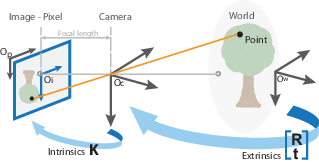Fig: Camera intrinsics and extrinsics relate (1) (Source: mathwork) In a short-word: extrinsics parameters help us turn world coordinates into camera coordinates. And intrinsic parameters help us turn camera coordinates into pixel coordinate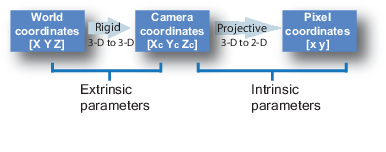Fig: Camera intrinsics and extrinsics relate (2) (Source: mathwork)

Example of Distortion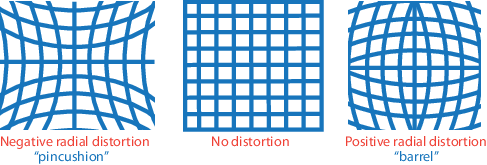Fig: Radial Distortion (Source: mathwork)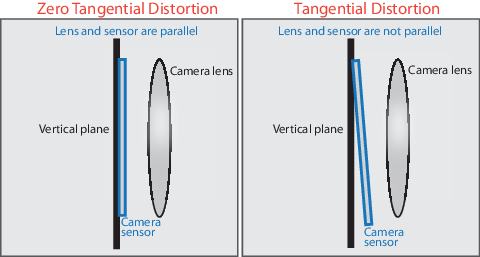Fig: Tangential Distortion (Source: mathwork)

# Camera Projection

Camera projection = convert 3d point (X, Y, Z) into camera’s pixel coordinate (x, y)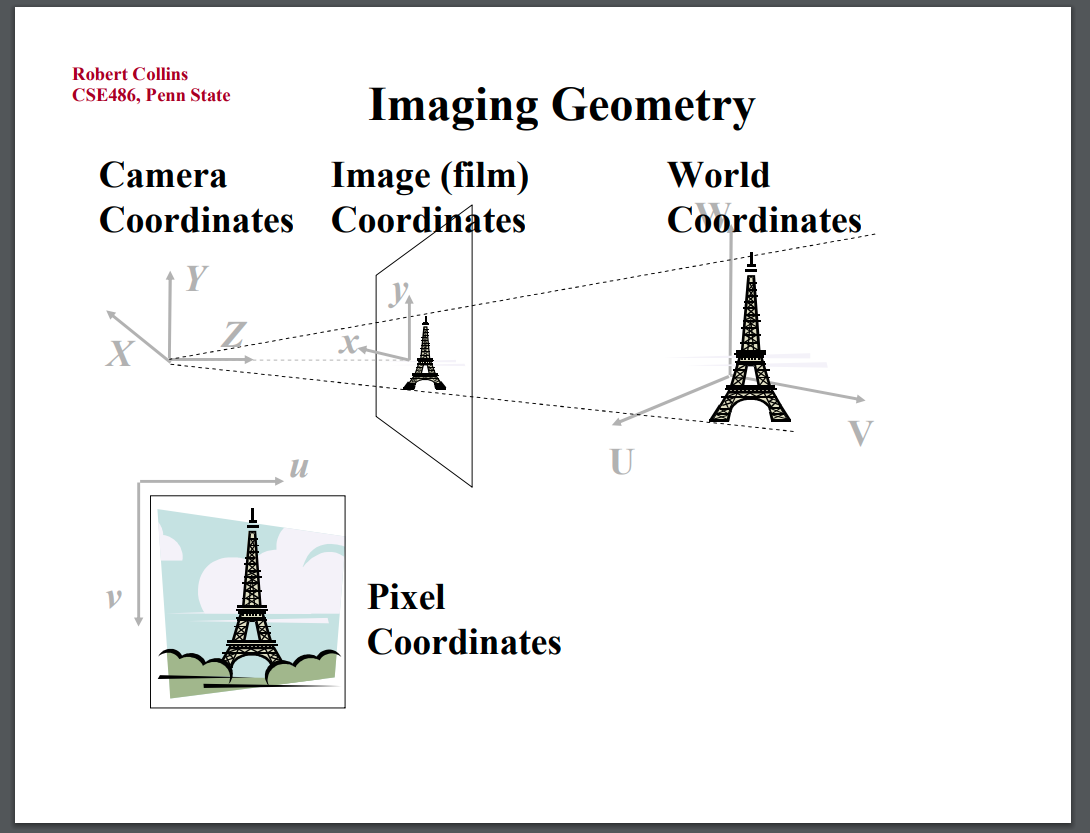Forward projection == convert World coords into pixel coords.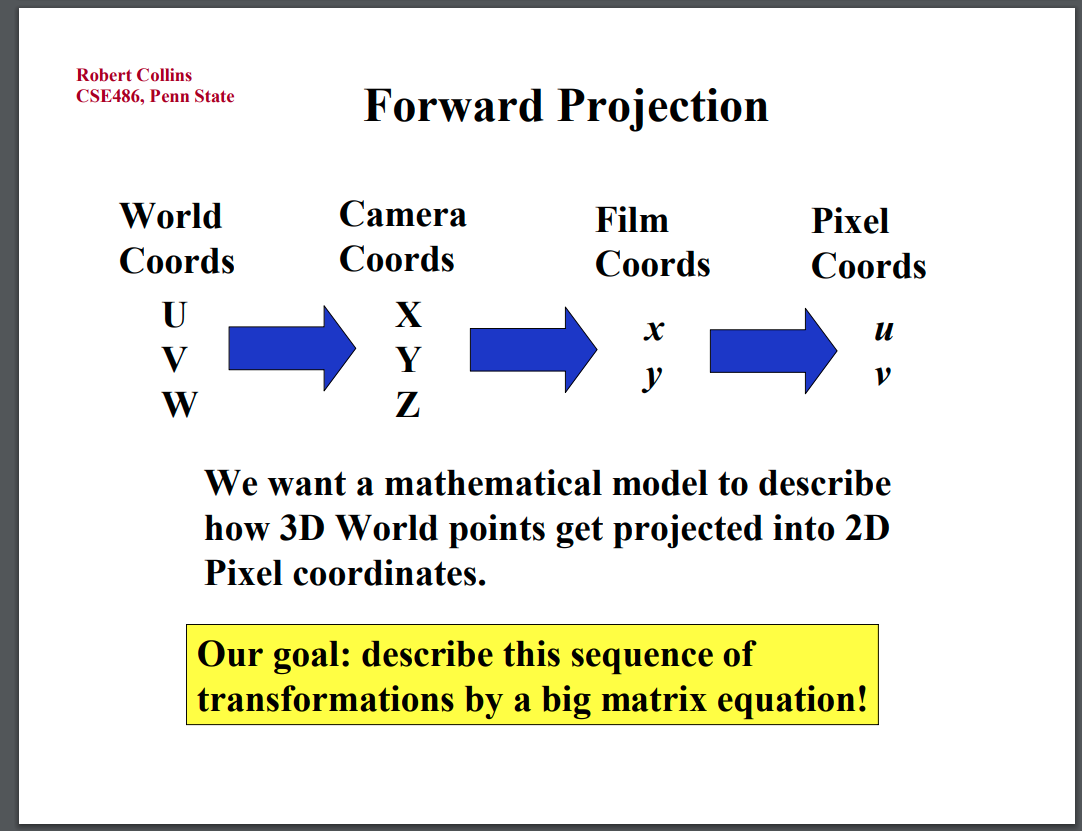Backward projection = convert pixel coords into world coords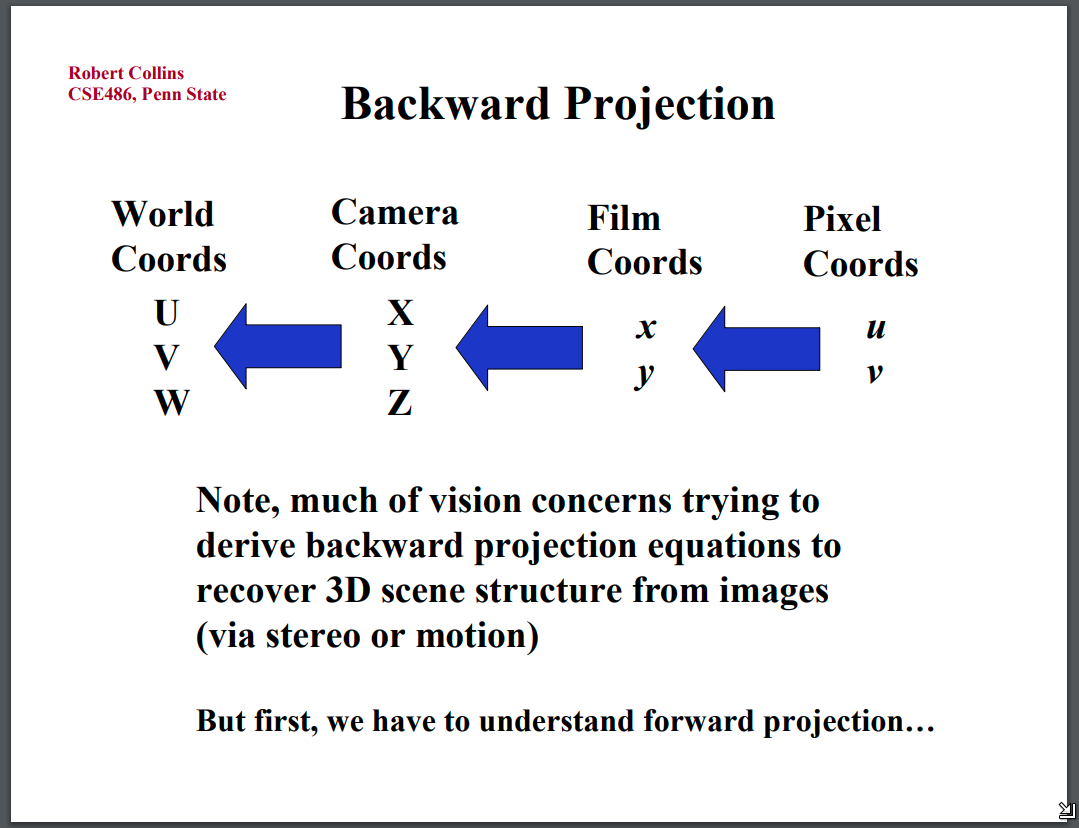The relation between foward projection and extrinsics (Mext) and extrinsics (Mint)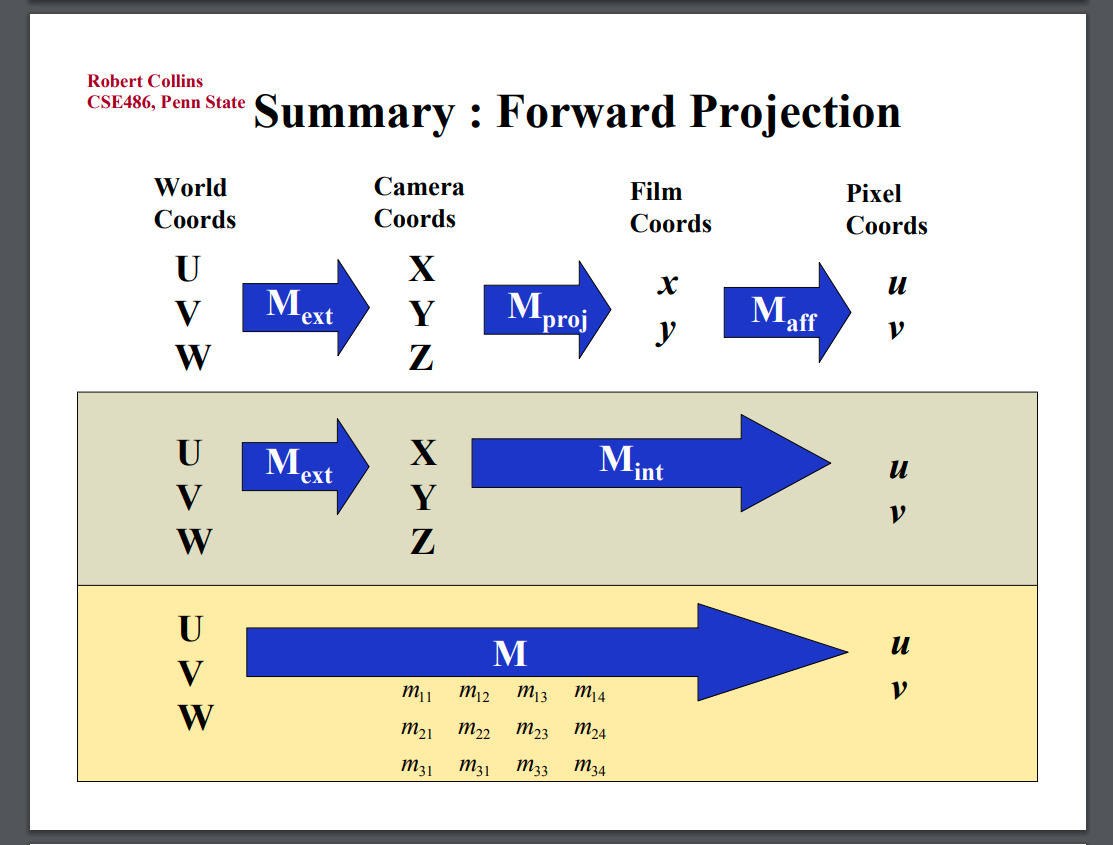In matrix manipulation format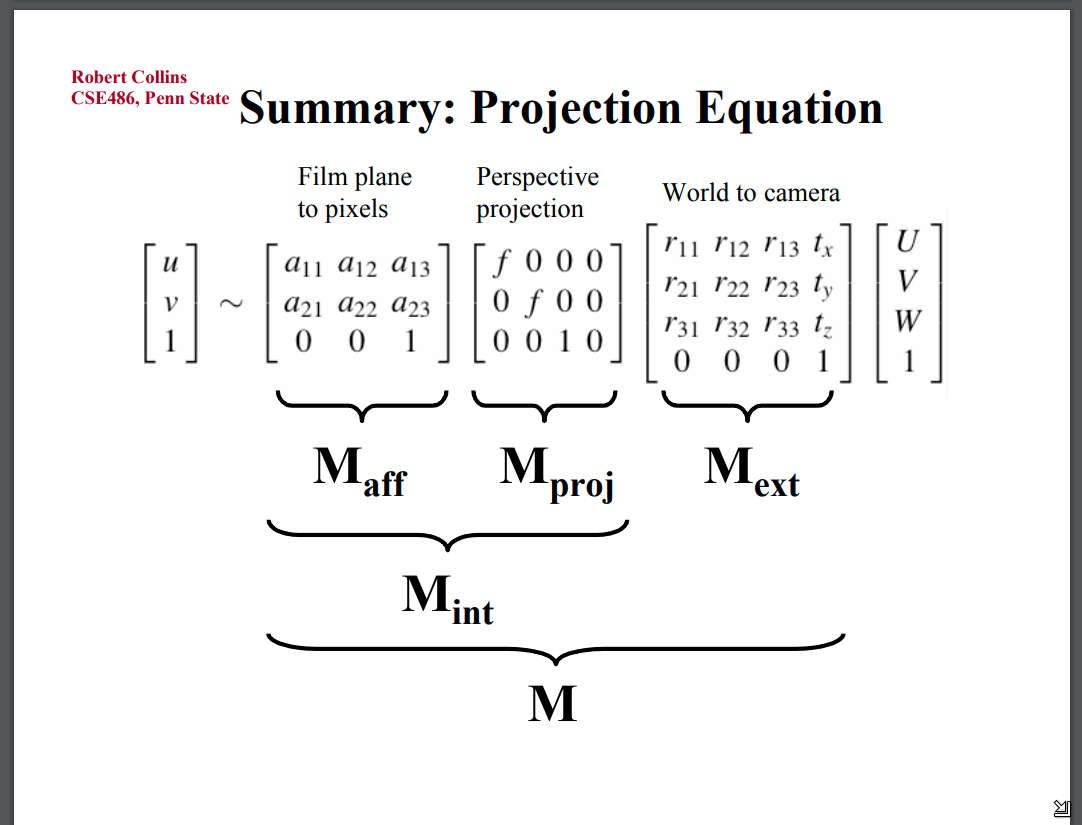## Camera extrinsics

The extrinsics is the result of a rotation and a translation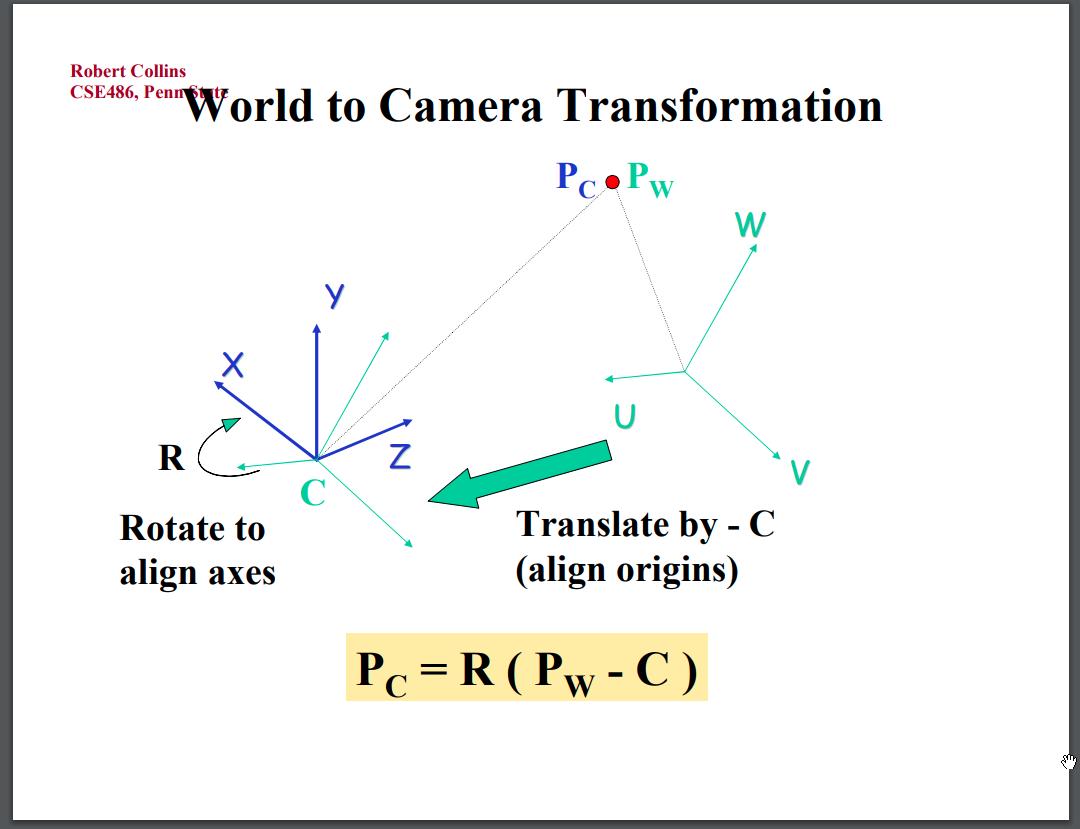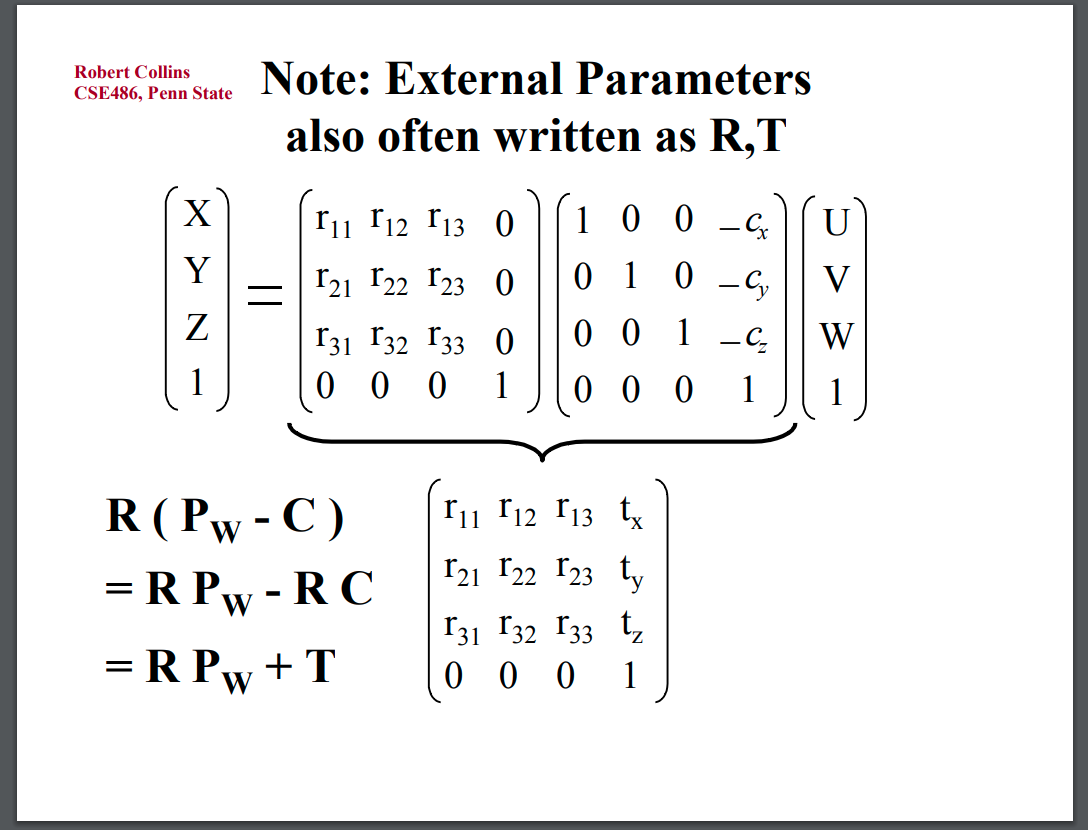## Camera intrinsics

The intrinsics is the result of a affine Transformation (Maff) and a perspective projection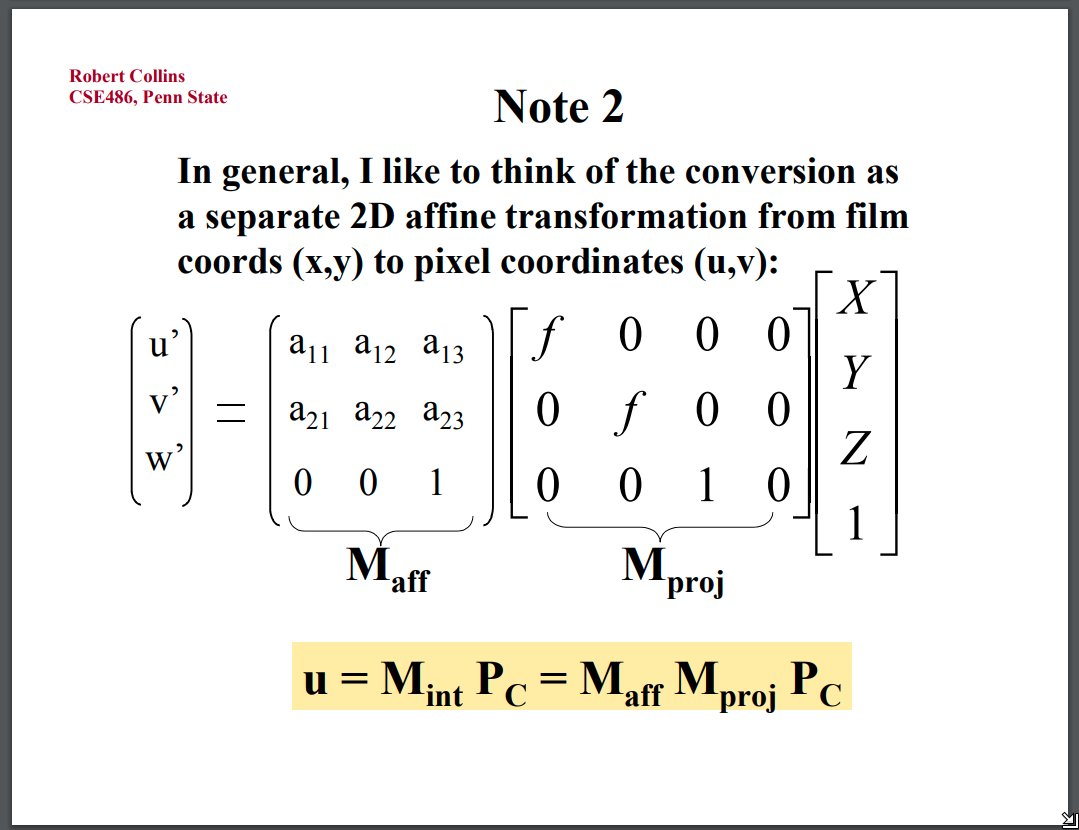The perspective projection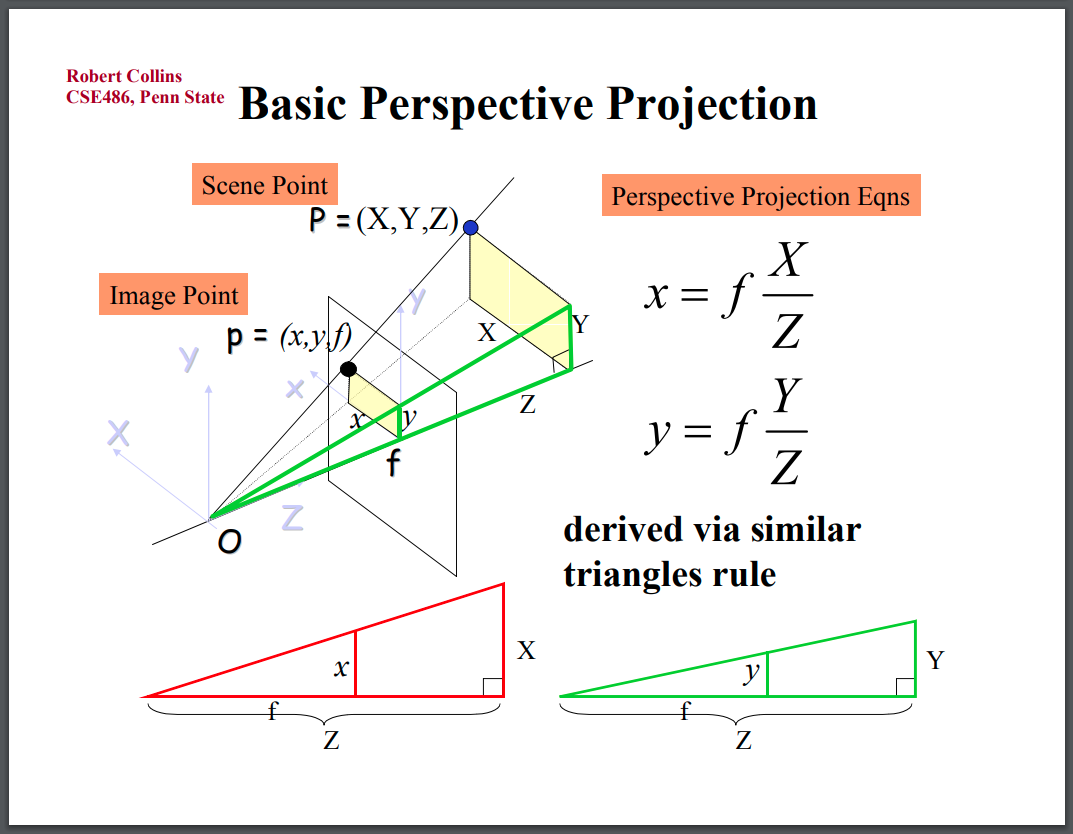Re-write in matrix manipulation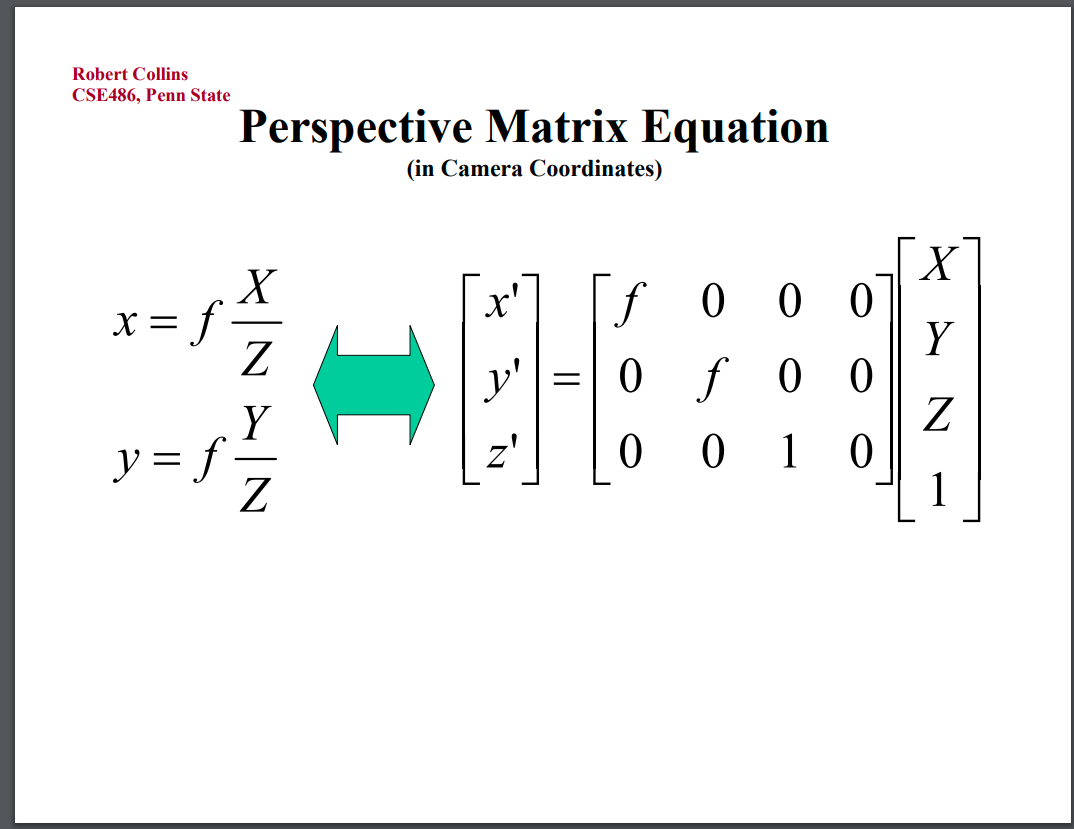The affine Transformation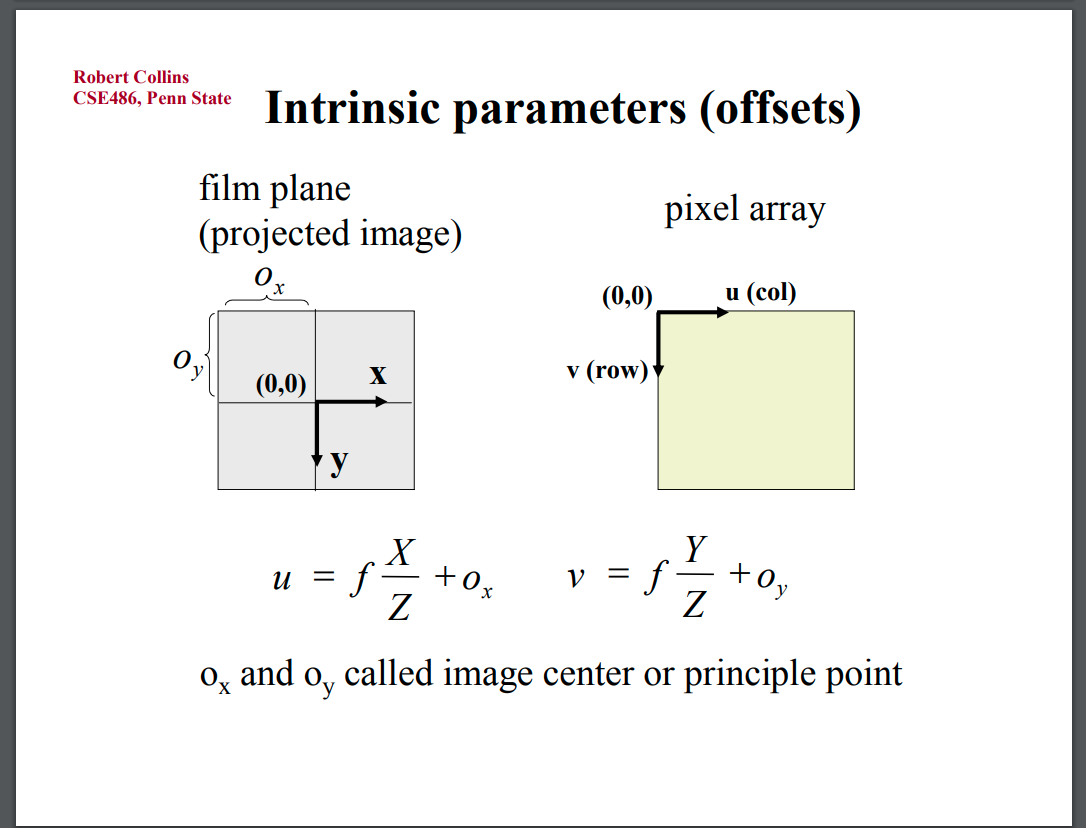The camera intrinsics is a 3x3 matrix as follow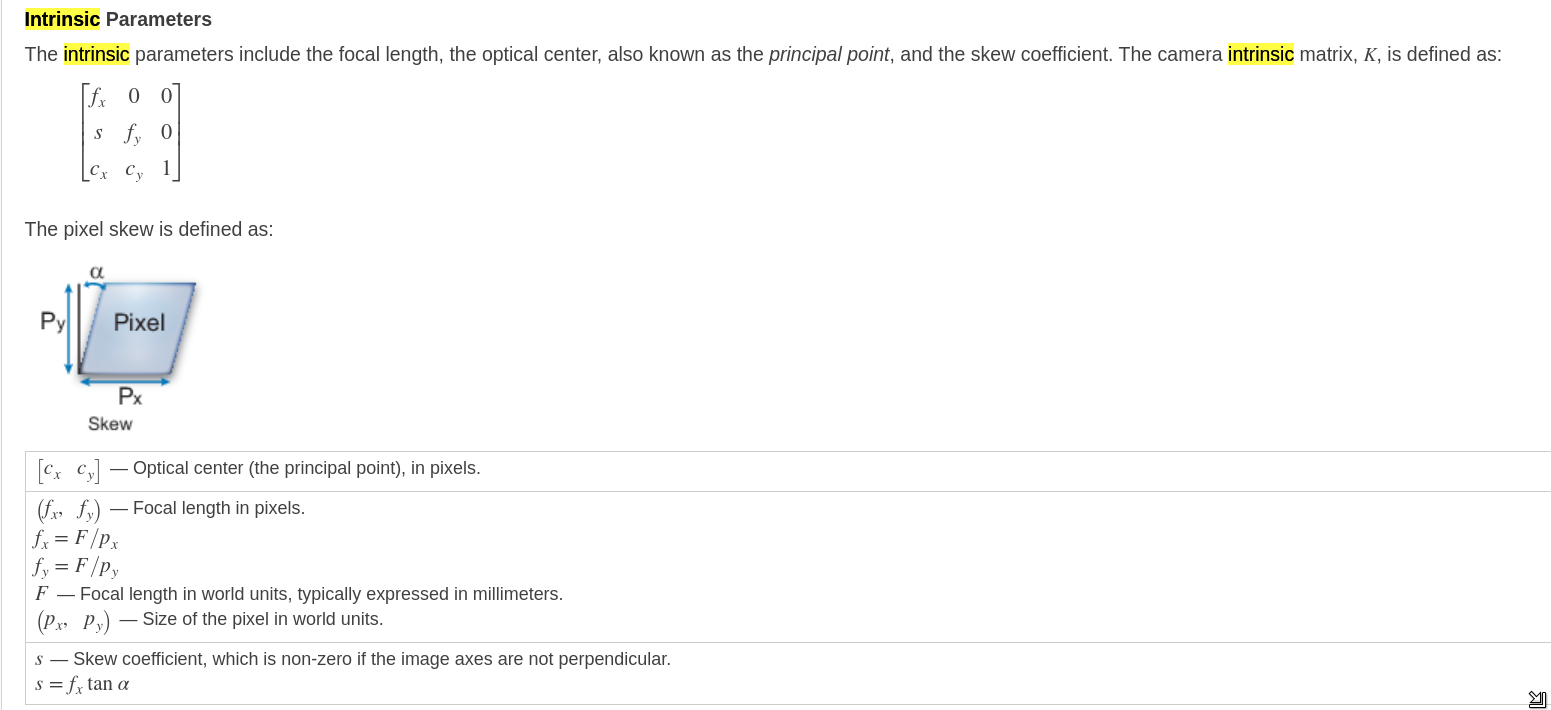# Camera Projection implementation

These code are taken from intel realsensen source code. For detail see https://github.com/IntelRealSense/librealsense/blob/master/include/librealsense2/rsutil.h

This is only used for check the matrix manipulation in above section

## Camera extrinsics

typedef struct rs2_extrinsics
{
float rotation;    /**< Column-major 3x3 rotation matrix */
float translation; /**< Three-element translation vector, in meters */
} rs2_extrinsics;


## Camera intrinsics

typedef struct rs2_intrinsics
{
int           width;     /**< Width of the image in pixels */
int           height;    /**< Height of the image in pixels */
float         ppx;       /**< Horizontal coordinate of the principal point of the image, as a pixel offset from the left edge */
float         ppy;       /**< Vertical coordinate of the principal point of the image, as a pixel offset from the top edge */
float         fx;        /**< Focal length of the image plane, as a multiple of pixel width */
float         fy;        /**< Focal length of the image plane, as a multiple of pixel height */
rs2_distortion model;    /**< Distortion model of the image */
float         coeffs; /**< Distortion coefficients */
} rs2_intrinsics;


or inside Ros’s senser_msgs. Please be noted that matlab use different format with ros.

# Intrinsic camera matrix for the raw (distorted) images.
#     [fx  0 cx]
# K = [ 0 fy cy]
#     [ 0  0  1]
# Projects 3D points in the camera coordinate frame to 2D pixel
# coordinates using the focal lengths (fx, fy) and principal point
# (cx, cy).
float64  K # 3x3 row-major matrix


## Camera projection

Pixel coords into Camera Coords

static void rs2_deproject_pixel_to_point(float point, const struct rs2_intrinsics * intrin, const float pixel, float depth)



Camera Coords into Pixel coords

static void rs2_project_point_to_pixel(float pixel, const struct rs2_intrinsics * intrin, const float point)


Camera 1 Coords -> World Coords -> Camera 2 Coords

static void rs2_transform_point_to_point(float to_point, const struct rs2_extrinsics * extrin, const float from_point)



End

Tags:

Categories:

Updated: